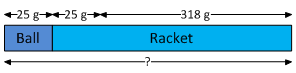### Sample Problem

The mass of a tennis ball is 25 g. It is 318 g lighter than a tennis racket. What is their total mass?

g

#### SolutionThe mass of the racket: 25 g + 318 g = 343 g

Thus, the total mass: 25 g + 343 g = 368 g